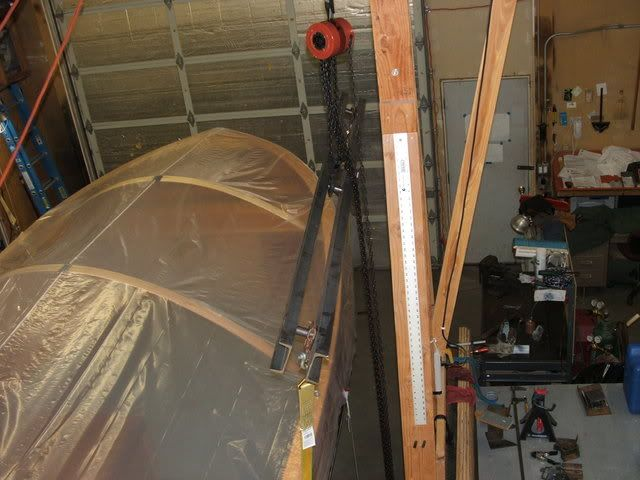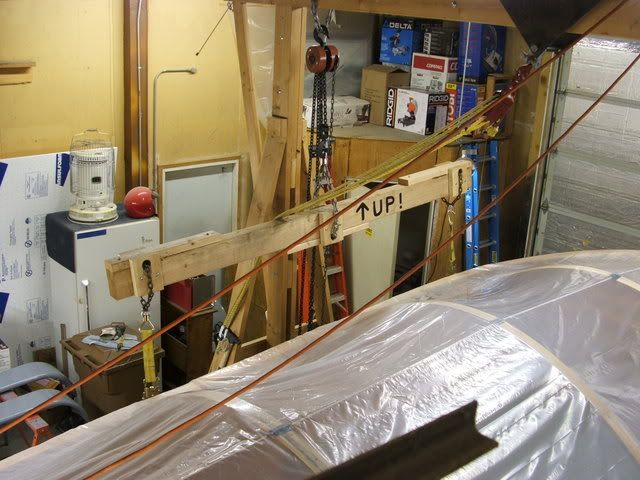## Is there an engineer in the house?

Topics not covered by another category.

Moderator: Bill Edmundson

Jones
Posts: 81
Joined: Mon Nov 17, 2003 11:22 am
Location: Idaho
Bill Edmundson wrote:P is you lifting force at the center. P/2 on each end. The distance from the end to the lift point is L/2. M= force x distance or P/2 x L/2 = PL/4

It's hard to believe that I made a living for almost 34 years on variations of one equation. Stress = P/A -+ MC/I.

Bill
Bill & FDMSIV,

Not to make this thread any more cumbersome but I really do need to understand this equation. Please address what parts I'm not following on:

I assume that the loads on the end of the beam are treated as if it were a simple cantilever force? (In two directions)

P= Force, which we are solving for in "reverse" because of the safety limitation to 10Kpsi?

I'm stabbing at this, combining both of your responses and reviewing the formulae as posted in this thread and this is where I'm getting lost, as per my question marks

FDMSIV: (Yield strength of material / safety factor) * 3.86 * 4 * (1 / 96) * 10000 (I'm assuming your original post of 1000 was a typo, as changing the value to 10K gave a sum of 1608 pounds, not 160.83 pounds)

Sectional Modulus * ? * ? * (?) * 10000 (Ok, you see I'm in trouble)

Where:

3.86 = 2(sec. mod. as posted in manuals)

4 = ? is this my 1/2 length expressed in feet?

(1/96) = ? The beam (overall) is expressed in inches? Why is it expressed as a fraction?

10,000 equals strength of material, reduced by a safety factor of five.

The reason I'm asking all these questions is because I need to be able to duplicate this on a fairly regular basis.
Avoid Haste.

Jones
Posts: 81
Joined: Mon Nov 17, 2003 11:22 am
Location: IdahoAvoid Haste.

Bill Edmundson
Posts: 11919
Joined: Wed Nov 23, 2005 6:45 am
Location: Birmingham, AL, USA
Contact:
We're looking at this as a simple beam only inverted.

P = the load at the center in pounds
L = length in inches 96"
fa = allowable stress = 10000 pounds per sq. in.
M = design bending moment in inch pounds
S = section modulus cubic inches = 3.86 in^3 (you had 2 C4x5.4's 1.93 each)

M = PL/4 =1608*96/4= 38592"#

fa = M/S = 38592/3.86 = 9997.9 psi

Bill
Mini -Tug, KH Tahoe 19 & Bartender 24 - There can be no miracle recoveries without first screwing up.
Tahoe 19 Build

Jones
Posts: 81
Joined: Mon Nov 17, 2003 11:22 am
Location: Idaho
To continue on the strength of material calculations part of this, here's a picture of a doug fir spreader I built:It's an 8' 4 x 6 (nominal) beam on a 7' actual spread and with your help I'd like to calculate the strength of this spreader. I want to do it by myself (sort of) so I can learn the process and be able to apply it in future applications. I'll start with what I can actually do by myselfActual beam cross section: 3.5 x 5.5 (in) so 19.25 sq.in.

Length between load points: 7.0', so 3.5' from center.

From Machinery's Handbook doug fir #2 = 1300 bending pounds per square inch (permissible working stresses).

After much editing:

P=4*fa*(S/L) where:

P= acceptable load for this device (solving for P, in other words)

fa = allowable stress (from text).

S = Sectional modulus (not quite able to get this exact number, still looking at Bill's figures)L= Length of span, total.

fa=1300, S=17.64, L= 84

Therefore:

4 x 1300 (17.64/84) = 1092 pounds limit for this spreader.

Hmmm... interesting, the doug fir spreader has about 2/3 the capacity of the steel one I built, but at a savings of about 80% (since steel has gone wild and I had to pay 75 cents/pound).
Last edited by Jones on Wed May 14, 2008 10:36 am, edited 2 times in total.
Avoid Haste.

Jones
Posts: 81
Joined: Mon Nov 17, 2003 11:22 am
Location: Idaho
Bill Edmundson wrote:We're looking at this as a simple beam only inverted.

P = the load at the center in pounds
L = length in inches 96"
fa = allowable stress = 10000 pounds per sq. in.
M = design bending moment in inch pounds
S = section modulus cubic inches = 3.86 in^3 (you had 2 C4x5.4's 1.93 each)

M = PL/4 =1608*96/4= 38592"#

fa = M/S = 38592/3.86 = 9997.9 psi

Bill
Hi Bill,

Thanks, the itemized description of variables is very helpful. In your example you've used P as a given (1608). How would the same equation look if solving for P rather than fa?
Avoid Haste.

Bill Edmundson
Posts: 11919
Joined: Wed Nov 23, 2005 6:45 am
Location: Birmingham, AL, USA
Contact:
1st throw one more in. S is really I/c.

I is bh^3/12 c= h/2 or S= bh^2/6

S= 3.5*(5.5)^2/6= 3.5(30.25)/6= 17.64

Playing with the equations

P=4*fa*S/L

Bill
Last edited by Bill Edmundson on Wed May 14, 2008 9:00 am, edited 1 time in total.
Mini -Tug, KH Tahoe 19 & Bartender 24 - There can be no miracle recoveries without first screwing up.
Tahoe 19 Build

Jones
Posts: 81
Joined: Mon Nov 17, 2003 11:22 am
Location: Idaho
Bill Edmundson wrote:1st throw one more in. S is really I/c.

I is bh^3/12 c= h/2 or S= bh^2/6

S= 3.5*(5.5)^2/6= 3.5(30.25)/6= 17.64

Playing with the equations

P= fa*S/L

BillOk... we're treating this as an inverted beam (yeah, you mentioned that, lol) as though it were hung from ends and a central load imposed, yes?

Ok, up to edit the post with the corrected S.

edit: And boy... does it look fouled up.
Avoid Haste.

Bill Edmundson
Posts: 11919
Joined: Wed Nov 23, 2005 6:45 am
Location: Birmingham, AL, USA
Contact:
P= 4* 1300*17.64/84= 1092#

I left out the 4 previously.That's why we have checkers!

Bill
Mini -Tug, KH Tahoe 19 & Bartender 24 - There can be no miracle recoveries without first screwing up.
Tahoe 19 Build

Jones
Posts: 81
Joined: Mon Nov 17, 2003 11:22 am
Location: Idaho
Bill Edmundson wrote:P= 4* 1300*17.64/84= 1092#

I left out the 4 previously.That's why we have checkers!

Bill
Got it! I had to enter it as 4*1300(17.64/84) on my TI for the language to be right, but I got the same sum. Thanks Bill, I've been needing to understand this for a while.

MJ
Avoid Haste.